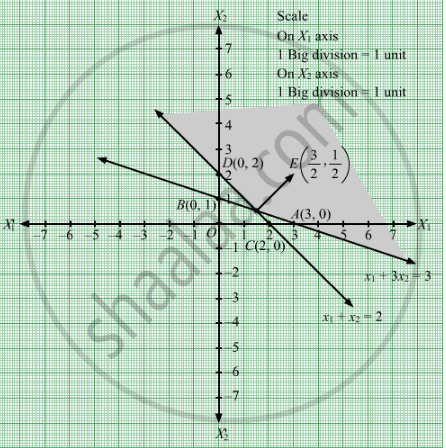# Minimize Z = 3x1 + 5x2 Subject to X 1 + 3 X 2 ≥ 3 X 1 + X 2 ≥ 2 X 1 , X 2 ≥ 0 - Mathematics

Sum

Minimize Z = 3x1 + 5x2
Subject to

$x_1 + 3 x_2 \geq 3$
$x_1 + x_2 \geq 2$
$x_1 , x_2 \geq 0$

#### Solution

First, we will convert the given inequations into equations, we obtain the following equations:
x1 + 3x2 = 3, x1 + x2 = 2, x1 = 0 and x2 = 0
Region represented by x1 + 3x2 ≥ 3 :
The line x1 + 3x2 = 3 meets the coordinate axes at A(3, 0) and B(0, 1) respectively. By joining these points we obtain the line x1 + 3x2 = 3.
Clearly (0,0) does not satisfies the inequation x1 + 3x2 ≥ 3 .So,the region in the plane which does not contain the origin represents the solution set of the inequation x1 + 3x2 ≥ 3.
Region represented by x1 + x2 ≥ 2:
The line x1 + x2 = 2 meets the coordinate axes at C(2, 0) and D(0, 2) respectively. By joining these points we obtain the line x1 + x2 = 2.Clearly (0,0) does not satisfies the inequation x1 + x2 ≥ 2. So,the region containing the origin represents the solution set of the inequation x1 + x2 ≥ 2.
Region represented by x1 ≥ 0 and x2 ≥ 0:
Since, every point in the first quadrant satisfies these inequations. So, the first quadrant is the region represented by the inequations x1 ≥ 0 and x2 ≥ 0.
The feasible region determined by the system of constraints, x1 + 3x2 ≥ 3 , x1 + x2 ≥ 2,x1 ≥ 0, and x2 ≥ 0, are as follows

.The corner points of the feasible region are O(0, 0), B(0, 1), $E\left( \frac{3}{2}, \frac{1}{2} \right)$  and C(2, 0).

The values of Z at these corner points are as follows.

Corner point Z = 3x1 + 5x2
O(0, 0) 3 × 0 + 5 × 0 = 0
B(0, 1) 3 × 0 + 5 × 1 = 5
$E\left( \frac{3}{2}, \frac{1}{2} \right)$
$\frac{3}{2}$ + 5 × $\frac{1}{2}$= 7

C(2, 0)
 3 × 2 + 5 × 0 = 6

Therefore, the minimum value of Z is 0 at the point O(0, 0). Hence, x1 = 0 and x2 = 0 is the optimal solution of the given LPP.
Thus, the optimal value of Z is 0.

Concept: Graphical Method of Solving Linear Programming Problems
Is there an error in this question or solution?

#### APPEARS IN

RD Sharma Class 12 Maths
Chapter 30 Linear programming
Exercise 30.2 | Q 16 | Page 32